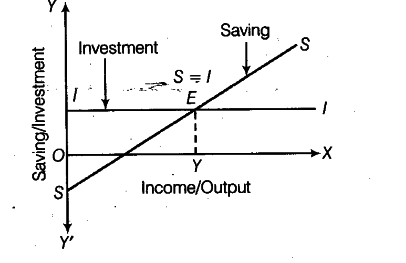# Explain with the help of a diagram,

Explain with the help of a diagram, how equilibrium level of income in an economy is determined by saving and investment curves? Will there always be full employment at equilibrium level of income?

Equilibrium level of income is determined at a point where ex-ante or planned saving is equal to planned investment. This is because, in equilibrium:
AS= AD or C + S = C +1 or S=I
Following figure illustrates the situation of equilibrium when S = IEquilibrium is struck at point E where S and I lines intersect each other. OY is the equilibrium level of income. There may or may not be a situation of full employment at the point of equilibrium. Because equilibrium simply refers to a situation when the desired level of saving (implying a leakage from the circular flow of income) is equal to the desired level of investment (implying an injection into the circular flow of income). This equality may or may not coincide with the situation of full employment in the economy.